# Class 6 Maths NCERT Solutions for Chapter 8 Decimals Ex – 8.5

## Decimals

Question 1.
Find the sum in each of the following:

(a) 0.007 + 8.5 + 30.08
(b) 15 + 0.632 + 13.8
(c) 27.076+ 0.55 + 0.004
(d) 25.65 + 9.005 + 3.7
(e) 0.75 + 10.425 + 2
(f) 280.69 + 25.2 + 38

Solution: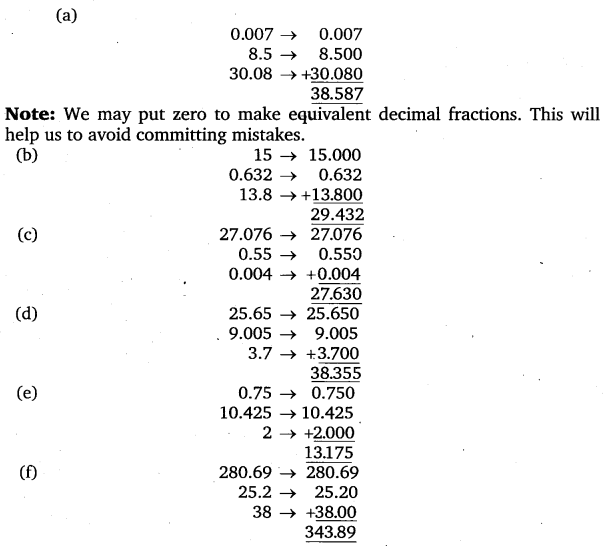Question 2.
Rashid spent ₹ 35.75 for Maths book and ₹ 32.60 for Science book. Find the total amount spent by Rashid.

Solution:
Money spent on Maths book = ₹ 35.75
Money spent on Science book=₹ 32.60
∴ Total money spent is =₹ 35.75 +₹ 32.60 = ₹ 68.35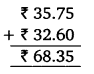Question 3.
Radhika’s mother gave her ₹ 10.50 and her father gave her ₹ 15.80, find the total amount given to Radhika by the parents.

Solution:
Money given by Radhika’s mother = ₹ 10.50
Money given by Radhika’s father = ₹ 15.80
∴ Total amount given to Radhika = ₹ 10.50 + ₹ 15.80 = ₹ 26.30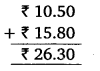Question 4.
Nasreen bought 3 m 20 cm cloth for her shirt and 2 m 5 cm cloth for her trouser. Find the total length of cloth bought by her.

Solution:
Cloth bought by Nasreen for her shirt = 3 m 20 cm
Cloth bought by Nasreen for her trouser = 2 m 5 cm
∴ Total cloth bought by Nasreen = 3 m 20 cm + 2 m 5 cm
= 5 m 25 cm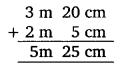Question 5.
Naresh walked 2 km 35 m in the morning and 1 km 7 m in the evening. How much distance did he walk in all?

Solution:
Distance walked in the morning = 2 km 35 m = 2.035 km
Distance walked in the evening = 1 km 7 m = 1.007 km
∴ Total distance walked by Naresh = 2.035 km +1.007 km = 3.042 km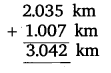Question 6.
Sunita travelled 15 km 268 m by bus, 7 km 7 m by car and 500 m on foot in order to reach her school. How far is her school from her residence?

Solution:
Distance travelled by bus = 15 km 268 m = 15.268 km
Distance travelled by car = 7 km 7 m = 7.007 km
Distance travelled on foot = 500 m = 0.500 km
Total distance of school from her residence
= 15.268 km + 7.007 km + 0.500 km = 22.775km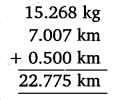Question 7.
Ravi purchased 5 kg 400 g rice, 2 kg 20 g sugar and 10 kg 850 g flour. Find the total weight of his purchases.

Solution:
Weight of rice purchased = 5 kg 400 g = 5.400 kg
Weight of sugar purchased = 2 kg 20 gm = 2.020 kg
Weight of flour purchased = 10 kg 850 g = 10.850 kg
Total weight of purchases = 5.400 kg +2.020 kg +10.850 kg = 18.270 kg